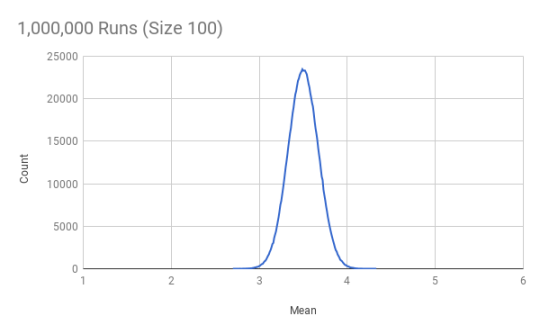# Last Uncertainty Wednesday we dug deeper into understanding the distribution of sample means. I…

Last Uncertainty Wednesday we dug deeper into understanding the distribution of sample means. I ended with asking why the chart for 100,000 samples of size 10 looked smoother than then one for samples of size 100 (just as a refresher, these are all rolls of a fair die). Well, for a sample of size 10, there are 51 possible values of the mean: 1.0, 1.1, 1.2, 1.3 … 5.8, 5.9, 6.0. But with a sample size of 100 there are 501 possible values for the mean. So with the same number of samples (100,000) the distribution will not be approximated as closely. We can fix this by upping the number of samples to say 1 million. Thanks to the amazing speed of a modern laptop even 100 million rolls of a die just take a couple of minutes (this still blows my. Here is the resulting chart:

Much smoother than before! We could make that even smoother by taking up the number of runs even further.

OK. So what would happen if we went to sample size 1,000? Well, by now this should be easy to predict. The distribution of sample means will be even tighter around 3.5 (the expected value of the distribution) and in order to get a smooth chart we have to further up the number of runs.

So what is the limit here? Well, this get us to the law of large numbers, which essentially states that the sample average will converge to the expected value as the sample grows larger. There is a strong version and a weak version of the law, a distinction which we may get to later (plus some more versions of the law).

For now though the important thing to keep in mind is that when we have small sample sizes, the sample mean may be far away from the expected value. And as we see above that even for a super simple probability distribution with 6 equally likely outcomes there is considerable variation in the sample mean even for samples of size 100! So it is very easy to make mistakes from jumping to conclusions on small samples.

Next Wednesday we will see that the situation is in fact much worse than that. Here is a hint: every sample has a mean (why?) but does every probability distribution have an expected value